# Radio Frequency (RF) Impedance Matching: Calculations and Simulations

### Abstract

This article explains the basics of radio frequency (RF) impedance matching, how to calculate the matching components, and how to check the results in LTspice®.

### Introduction

Electronic theory states that maximum power is transferred from a source to a load when the source resistance matches the load resistance. With most RF circuits, however, the source and load impedances have a reactive element, in which case the source impedance must be equal to the complex conjugate of the load impedance for maximum power transfer. In other words, while the real parts of the source and load impedance must match, the imaginary part of the load impedance must be opposite in sign to the imaginary part of the source impedance.

Much of the complexity of an RF power amplifier circuit is due to the impedance matching components surrounding the main active component, be that a transistor or integrated solution. Once it is understood how these RF impedance matching circuits have been calculated, the rest of the circuit is more straightforward. Calculating these matching components is a simple process, but sometimes it is good to have a tool to cross-check these calculations and ensure that, indeed, the maximum power is being transferred from the source to the load.

This article digs deeper into the impedance matching theory outlined in Chris Bowick’s book, RF Circuit Design, which many engineers regard as the radio frequency bible. Readers may want to refer to this book to get a fuller picture of other RF impedance matching networks. LTspice simulations are also included throughout the article to cross-check the calculations.

### Tuned Circuits and Loaded Q

A capacitor has a reactance given by Equation 1.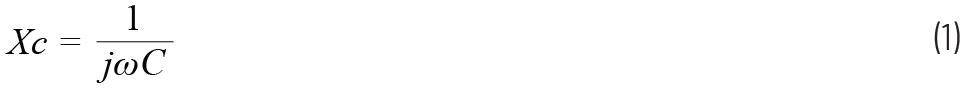Multiplying the top and bottom by j means that a capacitor has a negative reactance. In contrast, an inductor has a positive reactance given by Equation 2.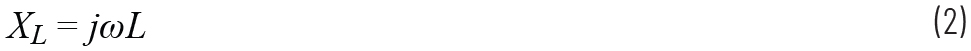Therefore, if we put a capacitor in series with an inductor and they both have equal but opposite reactances at one particular frequency, they form a short circuit with zero phase shift. Likewise, if these components are placed in parallel, they form an open circuit with zero phase shift: the net impedance of two components in parallel is found by dividing the product of the impedances by their sum and if they have equal but opposite reactances, the reactances create a zero-value denominator and thus an open circuit.

If a resistor is placed in parallel or series with a reactive component, then the loaded Q describes the ratio of the reactance to the resistance. The loaded Q of a parallel circuit is defined as:The loaded Q of a series circuit is defined as: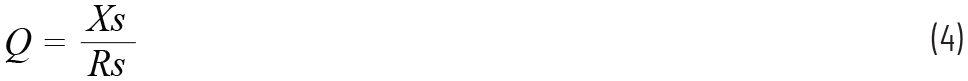Where Rp and Rs are the parallel and series resistors, and Xp and Xs are the parallel and series reactances.

We can use the loaded Q of a network to transform a parallel network to a series network and thus make the matching a lot easier. Once the series equivalent of a network is derived, it is then just a matter of choosing a source impedance with equal real, but opposite imaginary components to complete the impedance match.

The circuit shown in Figure 1 has an impedance given bywhere –jXcp is the reactance of the parallel capacitor.

The series equivalent of this circuit can be calculated in several ways. The top and bottom of Equation 5 can be multiplied by the complex conjugate of the denominator to obtain the real and imaginary series values. Alternatively, the equation can be converted to polar form and the real and imaginary series components can be calculated using sines and cosines.

A quicker way of obtaining the series components is to find the loaded Q (Qp) of the parallel network (using Equation 3), then use the equation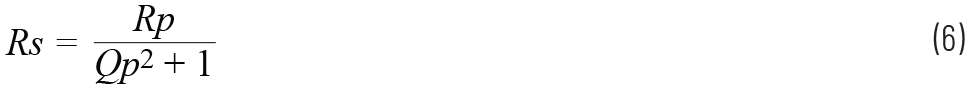to find the new series resistance. We then design a series network with the same Q and put the new series resistor, Rs, into Equation 4 to find the new series reactance, Xs. The derivation of Equation 6 is shown in the appendix.

Therefore, the circuit components in Figure 1 give a loaded Q of 10, which is found by dividing the parallel resistance by the parallel reactance. Using Equation 6, we can translate the parallel 1 kΩ resistor to a series resistor, Rs, of 9.9 Ω. We can then use Equation 4 to work out that the series capacitor needs to have a reactance of –j99 Ω. Thus, at a given frequency, the circuit in Figure 1 presents an identical impedance to the circuit in Figure 2.

Since the load has a real part of 9.9 Ω and a negative imaginary part (–j99 Ω), we need a source impedance with a real part of 9.9 Ω and a positive imaginary part (+j99 Ω) to ensure maximum power transfer will be achieved. In effect, by choosing a source reactance that is equal but opposite to the load reactance, these two reactances cancel each other out (creating a short circuit) and we are left with the source resistance driving an identical load resistance.

Now, the series circuit in Figure 2 is only an equivalent of the circuit in Figure 1. We don’t have to change the configuration of Figure 1. If we drive this parallel circuit with a source that has a series impedance of (9.9 + j99) Ω, maximum power will be transferred.

It is important to note that the parallel reactance (in this case, Cp) creates a lower value series equivalent of the 1 kΩ resistor by a factor determined by the Q of the circuit. This reactance can be either a series capacitor or a series inductor. The opposite is also true. In a series RC circuit (as shown in Figure 2), the series reactance (whether capacitive or inductive) creates a higher value parallel equivalent of the series resistor, Rs. Indeed, we can rearrange Equation 6 to readThis immediately shows us that the parallel resistance is a factor of (Q2 + 1) times higher than the series equivalent.

To summarize the previous example, we can use parallel or series reactances to make a resistor look smaller or larger. The loaded Q of the parallel RC network is determined by the ratio of its resistance to its reactance. We use this loaded Q to convert the parallel network into a series network and then choose the real part of the source impedance to equal the real part of the (series) load impedance, then choose a reactive part of the source impedance to be equal but opposite to the reactive part of the series load impedance.

#### Working with Fixed Source and Load Impedances

Unfortunately, we rarely have the luxury of being able to change the source impedance. The source and load impedances are normally predetermined, so we must design a network to match the two

Figure 3 shows a 50 Ω source that needs to be matched to a 1 kΩ load at 100 MHz. The shunt capacitor needs to transform the parallel 1 kΩ to a series 50 Ω resistor, which means this RC combination needs to have a loaded Q of 4.36, from Equation 6. This will give us an equivalent series resistance of 50 Ω plus a series capacitance of some value. We then choose a series inductor to create an equal but opposite reactance to the series capacitor. These two reactances cancel and we are left with a 50 Ω resistor feeding into a 50 Ω load.

We can use Equation 3 to calculate the reactance of the parallel capacitance. So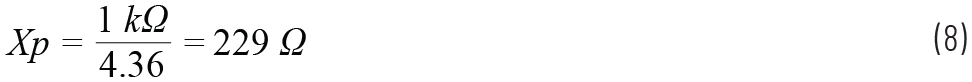The parallel capacitor should be 6.94 pF to present a reactance of –j229 Ω at 100 MHz. We now have a parallel network. To change this to a series network, we equate the Q’s of the parallel and series networks. Using Equation 4, we can see that this parallel circuit translates to a series equivalent network with Rs = 50 Ω and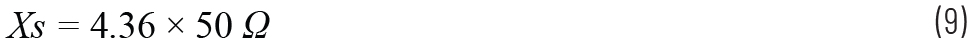So Xs = 218 Ω.

Therefore, the parallel combination of the 6.94 pF capacitor and the 1 kΩ load looks like a series 50 Ω resistor and a series capacitor with a reactance of –j218 Ω. Choosing a series inductor to give a +j218 Ω ensures the reactive parts of the matching network cancel and the 50 Ω source now feeds an effective load resistance of 50 Ω. Figure 4 shows the final network.

The circuit can be simulated in LTspice. The circuit is constructed as normal and for convenience the junction of R1 and L1 is labeled “IN.” The impedance of the load, as seen by the source, can be plotted by probing the IN node and the current flowing into L1. In the waveform window, right click over I(L1) and copy the text. Then right click over the V(in) icon and change the text to “V(in)/I(L1)” to plot the input impedance of the matching network, as shown in Figure 5.

However, the plot in Figure 5 shows the impedance in dB. To change the y-axes to show real and imaginary components, right click over the left-hand y-axis and change the Representation box from Bode to Cartesian, as shown in Figure 6.

The final plot is shown in Figure 7 with the real values plotted on the left axis and the imaginary values plotted on the right axis. Moving a cursor to the 100 MHz position, we can see that the input impedance of the matching network is indeed 50 Ω with very little imaginary component.

Unfortunately, if we close the waveform window and rerun the simulation, we need to re-probe V(in) and I(L1), divide one by the other, then change the y-axes to show the Cartesian values. This tedium can be avoided by selecting the waveform window and choosing File > Save Plot Settings As, as shown in Figure 8. This saves the setup of the current waveform screen and automatically re-plots the waveforms with the next simulation.

### Designing for a Known Q

In the previous example we used a parallel capacitor to make the 1 kΩ load resistor look like a 50 Ω series resistor plus some arbitrary series capacitor, then picked an inductor to cancel out the reactance of the series capacitor. This resulted in an L network, as shown in Figure 4. Unfortunately, the matching network in Figure 4 does not allow us to choose the Q—this is determined by the source and load impedances. One way of overcoming this is to use a T network, as shown in Figure 9, which consists of two back-to-back L networks.

In this example, we need to make the load resistance of 2.1 Ω look like 50 Ω while keeping the Q at a desired value. To do so, we really put the series to parallel conversion techniques to the test.

In the L network, we used a series inductor to cancel out the reactance of a series capacitor (to create a short circuit). In the T network, we also use a parallel inductor to cancel out the reactance of a parallel capacitor (to create an open circuit).

Looking at Figure 9, we know that the series impedance XS2 (whether a capacitor or inductor) translates the series 2.1 Ω load resistor to a larger equivalent parallel resistance, plus some arbitrary parallel reactance. Therefore, we can choose XP2 to be equal but opposite to this arbitrary parallel reactance and create an open circuit. We are then left with the 2.1 Ω resistor looking like a larger parallel resistor with no reactive element.

The 2.1 Ω load now looks like a much larger virtual resistor, Rv, as shown in Figure 9. Note that the resistor Rv is not actually placed in the circuit—we have merely made the 2.1 Ω resistor look like it has a larger value when viewed from the position of Rv. Indeed, to simplify the circuit, we could replace the 2.1 Ω resistor, XS2, and XP2 with Rv and the circuit will present the same impedance at one specific frequency when viewed from the source.

By rearranging Equation 6 to read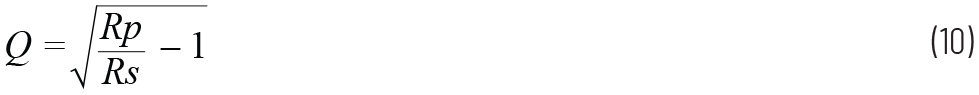We can see that the larger this virtual resistor is compared with the source resistor of 50 Ω, the larger is the circuit’s Q. Thus, we can modify XS2 and XP2 to make Rv look like any value and thus give us our desired circuit Q. We can then use the methodology used for an L network to match the source impedance (50 Ω) to the load resistor, Rv. We then use XP1 to make Rv look like a smaller series resistor (ideally 50 Ω), plus some arbitrary series reactance and we choose XS1 to be equal but opposite to this arbitrary series reactance, so we are left with the 50 Ω source resistance feeding into a 50 Ω load resistance, but still maintaining a high Q.

Let’s go through the methodology. We need to match the 50 Ω source to a 2.1 Ω load at 100 MHz with a desired circuit Q of 10. We are aiming to make the circuit of Figure 9 look like an L network with a 50 Ω source and a larger value load resistor, Rv. We first need to convert the 2.1 Ω to a higher value (parallel) resistor. We know that a series reactance, XS2, transforms the 2.1 Ω into a larger parallel resistance, so from Equation 4So XS2 = 21 Ω.

We then convert this series circuit to its parallel equivalent using Equation 6.So Rp is 212.1 Ω. This is our virtual resistor.

Therefore, a series resistance of 2.1 Ω and a series reactance of 21 Ω looks like a larger parallel resistor of 212.1 Ω plus some arbitrary parallel reactance. We then insert an equal but opposite parallel reactance to cancel this out to create an open circuit, so we are just left with our larger parallel resistance. To determine the parallel reactance, we use Equation 3, so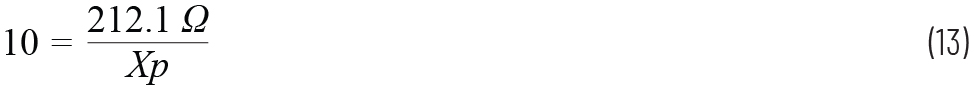So XP2 = 21.21 Ω.

If we wanted to, we could replace XS2, XP2, and the 2.1 Ω resistor with a single parallel resistor equal to 212.1 Ω and the circuit would present the same impedance to the source at 100 MHz. This can be simulated in LTspice. The circuit can now be treated as a simple L network where we are just matching the 50 Ω source resistor to a load of 212.1 Ω. From Equation 10,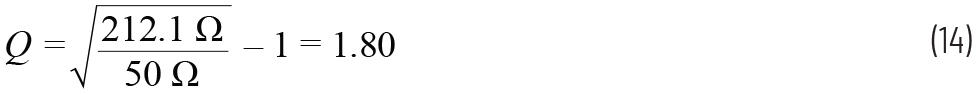We need to make this parallel 212.1 Ω resistor look like a series 50 Ω resistor. We know the resistance and we know the Q, so we can now work out the required parallel reactance to achieve this Q. We then create an equivalent series circuit with the same Q. Inserting a parallel reactance converts this high value parallel resistor into a lower value series resistor (of 50 Ω), plus some series reactance. From Equation 3,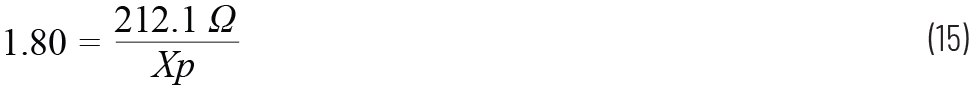So XP1 = 117.8 Ω.

So a parallel reactance of 117.8 Ω makes the parallel 212.1 Ω resistor look like a series resistor of 50 Ω. We can then use Equation 4 to calculate the series reactance.

The parallel reactance of 117.8 Ω and resistance of 212.1 Ω has a Q of 1.80. Converting this to a series circuit with the same Q and a series resistance of 50 Ω enables us to calculate the series reactance.

From Equation 4,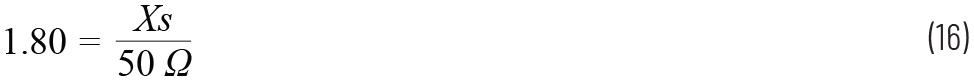The parallel resistance of 212.1 Ω and reactance of 117.8 Ω looks like a series resistance of 50 Ω plus reactance of 90 Ω. If XP1 is a capacitor, this translates to a series capacitance (of reactance –j90 Ω), hence the series matching component has to be inductive (of reactance +j90 Ω) so the reactances cancel.

There are a couple of points to note from the above procedure. Firstly, with a T network, the design starts at the end of the circuit with the lowest value resistor, which in the example above is the 2.1 Ω, not the 50 Ω. From Equation 6 we can see that, if we started with the 50 Ω end, we would calculate a much higher value for Rv and end up with a huge Q for the right half of the network due to the much lower load resistor of 2.1 Ω. Secondly, we notice that the series reactances always have the opposite sign to shunt reactance since we need to ensure that they cancel each other out. Hence series capacitors generally demand shunt inductors and vice versa.

The component values are shown in Table 1.

 Component Impedance Component Value at 100 MHz XS1 90 17.68 pF XP1 117.8 187.5 nH XS2 21 75.79 pF XP2 21.21 33.76 nH

Since XP1 and XP2 are parallel inductors, these can be combined into a single inductor with a value of 28.61 nH. The final circuit is shown in Figure 10.

Plotting the voltage at the IN node and dividing it by the current flowing into C1, we can see that the input impedance of the matching network is very nearly 50 Ω, as shown in Figure 11.

We can also plot the power transferred to the load by plotting the voltage across the load and the current flowing through the load and multiplying one by the other, as shown in Figure 12.

By right clicking over the plot window and selecting Add Plot Pane, we can simultaneously plot the matching in one window and the power transfer in another window. Saving the plot settings ensures these are plotted automatically after every simulation.

It is rare that the load is purely resistive. If the 2.1 Ω load in Figure 10 has a series capacitive element of, say, 398 pF (which equals –j4.0 Ω at 100 MHz), this disrupts the matching network. However, this is easy to overcome. In Figure 10, C2 and the 2.1 Ω load form a series network that has a Q of 10, determined by the ratio of the reactance (–j21 Ω) to the resistance (2.1 Ω). The additional –j4.0 Ω has increased the overall reactance to –j25 Ω. To maintain the same Q, we need to add a positive reactance to this series combination that cancels the –j4.0 Ω of the load. This can be achieved by adding an inductance of 6.37 nH (which gives a reactance of +j4.0 Ω) in series with C2 to maintain the ratio of the reactance to the resistance. A more convenient way is to increase the value of the capacitance C2 to 93.62 pF. This reduces the reactance to –j17 Ω and the load capacitance then increases the net series reactance back to –j21 Ω and thus a Q of 10 is maintained. LTspice can be used to provide a sanity check and the final circuit is shown in Figure 13.

Figure 14 shows this circuit has a good match to 50 Ω at 100 MHz with maximum power transferred.

### Conclusion

Hopefully this article has demystified the subject of RF impedance matching and made RF power amplifier circuits easier to understand. Using the loaded Q of a network, we can switch between series and parallel networks and translate low value resistors to higher value ones. However, as with any engineering task, it is always good to have a cross-check for the calculations and LTspice proves invaluable in plotting the input impedance and the power output of a circuit.

### 参考資料

Chris Bowick. RF Circuit Design. Newnes, November 2007.

### Appendix

The following equations reveal how we derived Equation 6.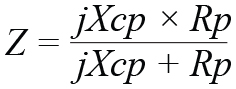To remove the imaginary term in the denominator: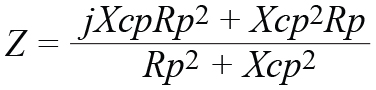Tidying up gives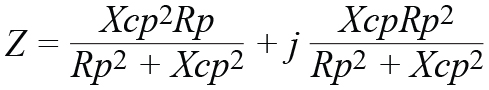The real part of the above is the value of our new series resistor, Rs. So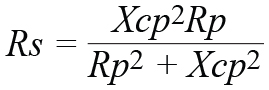From Equation 3 we know thatsoMultiplying by Qp2 and dividing by Rp2 gives